# Winter 2023 Midterm Exam

Instructor(s): Janine Tiefenbruck

This exam was administered in-person. The exam was closed-notes, except students were provided a copy of the DSC 10 Reference Sheet. No calculators were allowed. Students had 50 minutes to take this exam.

#### Video 🎥

Tropical cyclones, such as hurricanes, are storms characterized by high wind speeds. These storms can cause great devastation when they make landfall. In the US, the National Hurricane Center (NHC) is the government entity responsible for tracking and predicting these tropical weather systems. The NHC names tropical storms of sufficient intensity, using a list of people’s names that gets reused every six years. For example, there have been many different storms named Cindy, all occurring in different years. We’ll assume that no tropical storm has ever spanned more than one calendar year.

The DataFrame storms contains information about all named tropical storms in the Atlantic Ocean between 1965 and 2015. Each row corresponds to a data entry describing the storm at a particular moment in time, and one storm usually has many data entries throughout the duration of the storm. The columns of storms are as follows.

• "Name" (str): The name of the storm.
• "Date" (int): The year, month, and day of the data entry, stored as an 8-digit integer. For example, 20151113 corresponds to November 13, 2015.
• "Time" (str): The time of the data entry.
• "Status" (str): A categorical variable describing the intensity of the storm at the time of the data entry. There are many possible values, for example "TD" stands for “tropical depression” and "TS" stands for “tropical storm.”
• "Latitude" (str): The latitude of the center of the storm at the time of the data entry. Describes the north-south location of the storm.
• "Longitude" (str): The longitude of the center of the storm at the time of the data entry. Describes the east-west location of the storm.

A preview of storms is shown below.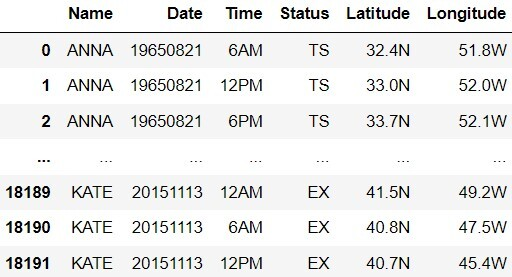Throughout this exam, we will refer to storms repeatedly. Assume that we have already run import babypandas as bpd and import numpy as np.

## Problem 1

### Problem 1.1

Which column would be an appropriate index for storms?

• 'Name'

• 'Date'

• 'Time'

• 'Latitude'

• None of these

An index should be unique. In this case 'Name', 'Date', 'Time', and 'Latitude' have repetative values, which does not make them unique. Remember 'Name' will be reused every six years, multiple hurricanes could happen on the same date, time, or latitude.

##### Difficulty: ⭐️⭐️⭐️

The average score on this problem was 69%.

## Problem 2

### Problem 2.1

The "Date" column stores the year, month, and day together in a single 8-digit int. The first four digits represent the year, the next two represent the month, and the last two represent the day. For example, 19500812 corresponds to August 12, 1950. The get month function below takes one such 8-digit int, and only returns the month as an int. For example, get month(19500812) evaluates to 8.

def get_month(date):
return int((date % 10000) / 100)

Similarily, the get year and get day functions below should each take as input an 8-digit int representing a date, and return an int representing the year and day, respectively. Choose the correct code to fill in the blanks.

def get_year(date):
return ____(a)____
def get_day(date):
return ____(b)____

What goes in blank (a)?

• date / 10000

• int(date / 10000)

• int(date % 10000)

• int((date % 10000) / 10000)

Answer: int(date/10000)

The problem is asking us to find the code for blank (a) and get_year is asking us to find the year. Let’s use 19500812 as an example, so we need to convert 19500812 to 1950. If we plug in Option 2 for (a) we will get 1950. This is because \frac{19500812}{10000} = 1950.0812, and when int() is applied to 1950.0812 then it will drop the decimal, which returns 1950.

Option A: date / 10000 If we plugged in Option 1 into blank (a) we would get: \frac{19500812}{10000} = 1950.0812. This is a float and is not equal to 1950.

Option C: int(date % 10000) If we plugged in Option 3 into blank (a) we would get: 812. Remember, % is the operation to find the remainder. We can manually find the remainder by doing \frac{19500812}{10000} = 1950.0812, then looking at the decimal, and noticing 812 cannot be divided by 10000 evenly. This is an int, but not the year.

Option D: int((date % 10000) / 10000) If we plugged in Option 4 into blank (a) we would get: 0.0812. We get this number by once again looking at the remainder of \frac{19500812}{10000} = 1950.0812, which is 812, and then dividing 812 by 10000. This is a float and is not equal to 1950.

##### Difficulty: ⭐️⭐️⭐️

The average score on this problem was 67%.

### Problem 2.2

What goes in blank (b)?

• int(date / 100)

• int(date / 1000000)

• int((date % 100) / 10000)

• date % 100

storms = storms.assign(Year = storms.get("Date").apply(get_year),
Month = storms.get("Date").apply(get_month),
Day = storms.get("Date").apply(get_day))

Answer: date % 100

The problem is asking us to find the code for blank (b) and get_day is asking us to get the day. Let’s use 19500812 as the example again, so we need to convert 19500812 to 12. Remember, % is the operation to find the remainder. If we plug in Option 4 for (b) we will get 12. This is because \frac{19500812}{100} = 195008.12 and by looking at the decimal place we notice 12 cannot be divided by 100 evenly, making the remainder 12.

Option 1: int(date / 100) If we plugged in Option 1 into blank (b) we would get 195008. This is because Python would do: \frac{19500812}{100} = 195008.12 then would drop the decimal due to the int() function. This is not the day.

Option 2: int(date / 1000000) If we plugged in Option 2 into blank (b) we would get 19. This is because Python would do: \frac{19500812}{1000000} = 19.500812 then would drop the decimal due to the int() function. This is a day, but not the one we are looking for.

Option 3: int((date % 100) / 10000) If we plugged in Option 3 into blank (b) we would get 0. This is because Python works from the inside out, so it will first evaluate the remainder: \frac{19500812}{100} = 195008.12, by looking at the decimal place we notice 12 cannot be divided by 100 evenly, making the remainder 12. Python then does \frac{12}{10000} = 0.0012. Remember that int() drops the decimal, so by plugging a date into this code it will return 0, which is not a day.

##### Difficulty: ⭐️⭐️⭐️

The average score on this problem was 56%.

Important! For the rest of the exam, assume those three functions have been implemented correctly and the following code has been run to assign three new columns to storms.

## Problem 3

### Problem 3.1

The DataFrame amelia was created by the code below, and is shown in its entirety.

amelia = (storms[(storms.get("Name") == "AMELIA") &
(storms.get("Year") == 1978)]
.get(["Year", "Month", "Day", "Time",
"Status", "Latitude", "Longitude"]))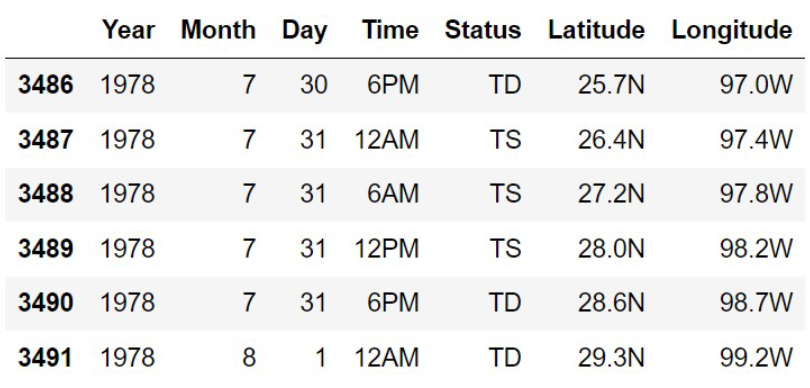Use the space provided to show the DataFrame that results from

        amelia.groupby("Status").max()

The column labels should go in the top row, and the row labels (index) should go in the leftmost row. You may not need to use all the rows and columns provided.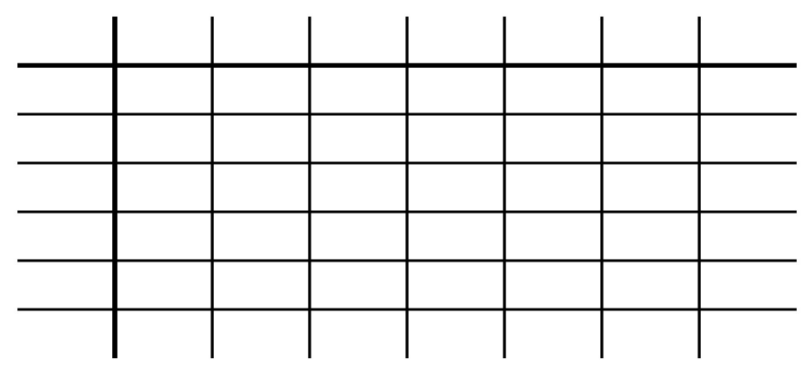Status Year Month Day Time Latitude Longtitude
TD 1978 8 31 6pm 29.3N 99.2W
TS 1978 7 31 6am 28.0N 98.2W

Remember that calling .groupby('column name') sets the column name to the index. This means the 'Status' column will become the new bolded index, which is found in the leftmost column of the data frame. Another thing to know is Python will organize strings in the index in alphabetical order, so for example TS and TD both start with T, but D comes sooner in the alphabet than S, which makes row TD come before row TS.

Next it is important to look at the .max(), which tells us that we want the maximum element of each column that correspond to the unique 'Status'. Recall how .max() interacts with strings: .max() will organize strings in the columns in descending order, so the last alphabetically/numerically.

##### Difficulty: ⭐️⭐️⭐️

The average score on this problem was 59%.

## Problem 4

### Problem 4.1

Suppose there are n different storms included in storms. Say we create a new DataFrame from storms by adding a column called 'Duration' that contains the number of minutes since the first data entry for that storm, as an int. The first few rows of this new DataFrame are shown below.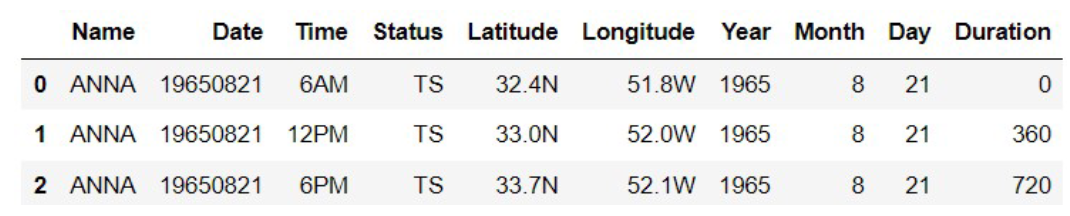Next we sort this DataFrame in ascending order of 'Duration' and save the result as storms_by_duration. Which of the following statements must be true? Select all that apply.

• The first n rows of storms_by_duration will all correspond to different storms, because they will contain the first reading from each storm in the data set.

• The last n rows of storms_by_duration will all correspond to different storms, because they will contain the last reading from each storm in the data set.

• storms_by_duration will contain exactly n rows.

• len(storms_by_duration.take(np.arange(n)).get("Name").unique()) will evaluate to n.

Answer: “The first n rows of storms_by_duration will all correspond to different storms, because they will contain the first reading from each storm in the data set.”

Let’s first analyze the directions. According to the directions, we added the column 'Duration', so we know how long each storm lasted. Then we sorted the DataFrame in ascending order, which will put the storms with the shortest duration at the top.

Each row will be tied to a unique storm because each storm can only have one minimum. This means storms_by_duration’s first n rows will contain the shortest duration for each unique storm, which corresponds to the first option.

Option 2: This is incorrect because even though the DataFrame is sorted in ascending order it is possible for a storm to have multiple close values in 'Duration', which does not guarantee unique storms in the last n rows. For example if you had the storm 'alice', which one time had a duration of 60 and the longest duration of 62. The values will be sorted such that 60 will come before 62, but they are within the last n values of the DataFrame, causing 'alice' to appear twice.

Option 3: This is incorrect because there can be more than n rows. It is possible that a storm appears multiple times. For example the storm Anna occured three different times on August 21, 1965 without sorting.

Option 4: This is incorrect. The code written will take the first n rows of the table, get the names, and find the number of unique named storms. Names are not unique, so it is possible for the storms to share the same name. This can be seen in the DataFrame example above.

##### Difficulty: ⭐️⭐️⭐️

The average score on this problem was 66%.

## Problem 5

### Problem 5.1

Latitude measures distance from the equator in the North or South direction. Longitude measures distance from the prime meridian in the East or West direction.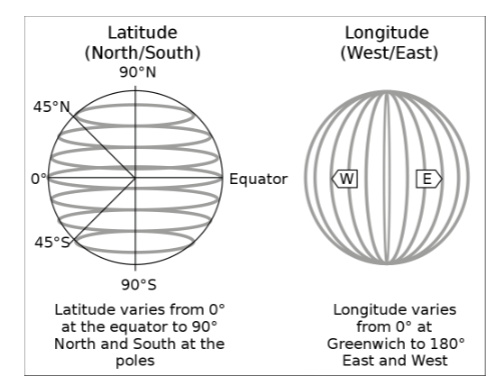Since all of the United States lies north of the equator and west of the prime meridian, the last character of each string in the "Latitude" column of storms is "N", and the last character of each string in the "Longitude" column is "W". This means that we can refer to the latitude and longitude of US locations by their numerical values alone, without the directions "N" and "W". The map below shows the latitude and longitude of the continental United States in numerical values only.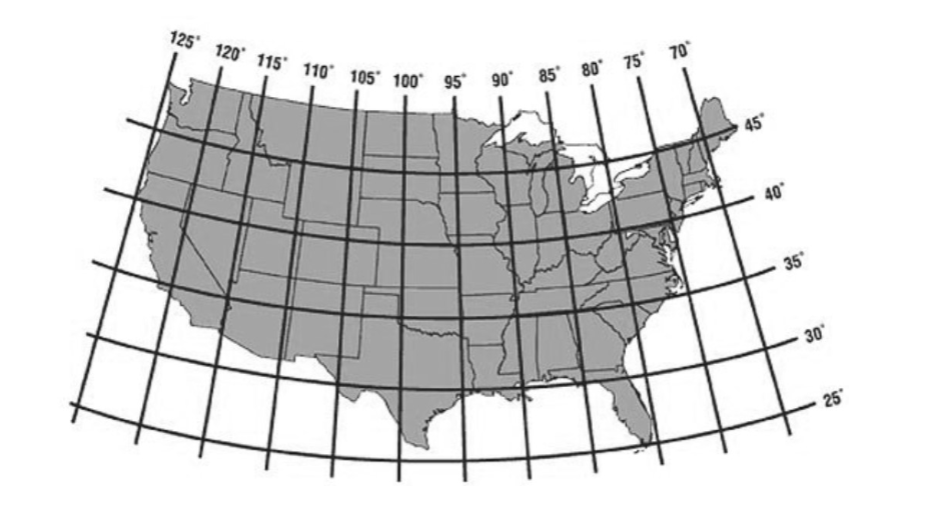1. Complete the function lat_long_numerical that takes as input a string representing a value from either the "Latitude" or "Longitude" column of storms, and returns that latitude or longitude as a float. For example, lat_long_numerical(34.1N) should return 34.1.
    def lat_long_numerical(lat_long):
return ________

Hint: The string method .strip() takes as input a string of characters and removes all instances of those characters at the beginning and end of the string. For example, "www.dsc10.com".strip("cmowz.") evaluates to "dsc10".

What goes in the blank? To earn credit, your answer must use the string method: .strip().

Answer: float(lat_long.strip("NW"))

According to the hint, .strip() takes as input of a string of characters and removes all instances of those characters at the beginning or the end of the string. The input we are given, lat_long is the latitude and longitude, so we able to take it and use .strip() to remove the "N" and "W". However, it is important to mention the number we now have is still a string, so we put float() around it to convert the string to a float.

##### Difficulty: ⭐️⭐️⭐️

The average score on this problem was 70%.

### Problem 5.2

Assume that lat_long_numerical has been correctly implemented. Which of the following correctly replaces the strings in the 'Latitude' and 'Longitude' columns of storms with float values? Select all that apply.

Option 1:

    lat_nums = storms.get('Latitude').apply(lat_long_numerical)
long_num = storms.get('Longitude').apply(lat_long_numerical)
storms = storms.drop(columns = ['Latitude', 'Longitude'])
storms = storms.assign(Latitude = lat_num, Longitude = long_num)

Option 2:

    lat_nums = storms.get('Latitude').apply(lat_long_numerical)
long_num = storms.get('Longitude').apply(lat_long_numerical)
storms = storms.assign(Latitude = lat_num, Longitude = long_num)
storms = storms.drop(columns = ['Latitude', 'Longitude'])

Option 3:

    lat_nums = storms.get('Latitude').apply(lat_long_numerical)
long_num = storms.get('Longitude').apply(lat_long_numerical)
storms = storms.assign(Latitude = lat_num, Longitude = long_num)
• Option 1

• Option 2

• Option 3

Answer: Option 1 and Option 3

Option 1 is correct because it applies the function lat_long_numerical to the 'Latitude' and 'Longitude' columns. It then drops the old 'Latitude' and 'Longitude' columns. Then creates new 'Latitude' and 'Longitude' columns that contain the float versions.

Option 3 is correct because it applies the function lat_long_numerical to the 'Latitude' and 'Longitude' columns. It then re-assigns the 'Latitude' and 'Longitude' columns to the float versions.

Option 2 is incorrect because it re-assigns the 'Latitude' and 'Longitude' columns to the float versions and then drops the 'Latitude' and 'Longitude' columns from the DataFrame.

##### Difficulty: ⭐️⭐️

The average score on this problem was 86%.

Important! For the rest of the exam, assume that the 'Latitude' and 'Longitude' columns of storms now have numerical entries.

## Problem 6

### Problem 6.1

Storm forecasters are very interested in the direction in which a tropical storm moves. This direction of movement can be determined by looking at two consecutive rows in storms that correspond to the same storm.

The function direction takes as input four values: the latitude and longitude of a data entry for one storm, and the latitude and longitude of the next data entry for that same storm. The function should return the direction in which the storm moved in the period of time between the two data entries. The return value should be one of the following strings:

• "NE" for Northeastern movement,
• "NW" for Northwestern movement,
• "SW" for Southwestern movement, or
• "SE" for Southeastern movement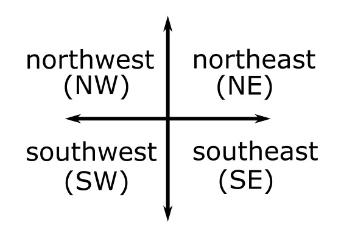For example, direction(23.1, 75.1, 23.4, 75.7) should return "NW". If the storm happened to move directly North, South, East, or West, or if the storm did not move at all, the function may return any one of "NE", "NW", "SW", or "SE". Fill in the blanks in the function direction below. It may help to refer to the images on Page 5.

def direction(old_lat, old_long, new_lat, new_long):
if old_lat > new_lat and old_long > new_long:
return ____(a)____
elif old_lat < new_lat and old_long < new_long:
return ____(b)____
elif old_lat > new_lat:
return ____(c)____
else:
return ____(d)____

What goes in blank (a)?

• "NE"

• "NW"

• "SW"

• "SE"

Answer: "SE"

According to the given example that direction(23.1, 75.1, 23.4, 75.7) should return "NW", we learn that having an old_lat < new_lat (in the example: 23.1 < 23.4) causes for the storm to move North and that having a old_long < new_long (in the example: 75.1 < 75.7) causes for the storm to move West. This tells us if old_lat > new_lat then the storm will move South, the opposite direction of North, and if old_long > new_long then the storm will move East, the opposite direction of West.

The if statement is looking for the direction where (old_lat > new_lat and old_long > new_long), so from above we can tell it is moving Southeast. Remember both conditions must be satisfied for this if statement to execute.

##### Difficulty: ⭐️⭐️⭐️

The average score on this problem was 73%.

### Problem 6.2

What goes in blank (b)?

• "NE"

• "NW"

• "SW"

• "SE"

Answer: "NW"

Recall from the previous section the logic that:

• North: old_lat < new_lat
• South: old_lat > new_lat
• East: old_long > new_long
• West: old_long < new_long

This means we are looking for the directions that satisfy the elif statement: old_lat < new_lat and old_long < new_long. Looking at our logic the statement is satisfied by the direction Northwest.

##### Difficulty: ⭐️⭐️

The average score on this problem was 79%.

### Problem 6.3

What goes in blank (c)?

• "NE"

• "NW"

• "SW"

• "SE"

Answer: "SW"

We know the answers cannot be 'SE' or 'NW' because the if statement and elif statement above the one we are currently working in will catch those. This tells us we are either working with 'SW' or 'NE'. From the logic we established in the previous subparts we know when 'old_lat' > 'new_lat' we know the storm is going in the Southern direction. This means the only possible answer is 'SW'.

##### Difficulty: ⭐️⭐️⭐️

The average score on this problem was 65%.

### Problem 6.4

What goes in blank (d)?

• "NE"

• "NW"

• "SW"

• "SE"

Answer: "NE"

The only option we have left is 'NE'. Remember than Python if statements will run every single if, and if none of them are triggered then it will move to the elif statements, and if none of those are triggered then it will finally run the else statement. That means whatever direction not used in parts a, b, and c needs to be used here.

##### Difficulty: ⭐️⭐️⭐️

The average score on this problem was 61%.

## Problem 7

### Problem 7.1

The most famous Hurricane Katrina took place in August, 2005. The DataFrame katrina_05 contains just the rows of storms corresponding to this hurricane, which we’ll call Katrina’05.

Fill in the blanks in the code below so that direction_array evaluates to an array of directions (each of which is"NE", "NW", "SW", or "SE") representing the movement of Katrina ’05 between each pair of consecutive data entries in katrina_05.

    direction_array = np.array([])
for i in np.arange(1, ____(a)____):
w = katrina_05.get("Latitude").____(b)____
x = katrina_05.get("Longitude").____(c)____
y = katrina_05.get("Latitude").____(d)____
z = katrina_05.get("Longitude").____(e)____
direction_array = np.append(direction_array, direction(w, x, y, z))

What goes in blank (a)?

Answer: katrina_05.shape

In this line of code we want to go through the entire Katrina’05 DataFrame. We are provided the inclusive start, but we need to find the exclusive stop, which would be the number of rows in the DataFrame. katrina_05.shape takes the DataFrame, finds the shape, which is represented by: (rows, columns), and then isolates the rows in the first index.

##### Difficulty: ⭐️⭐️⭐️⭐️

The average score on this problem was 46%.

### Problem 7.2

What goes in blank (b)?

Answer: iloc[i-1]

In this line of code we want to find the latitude of the row we are in. The whole line of code: katarina_05.get("Latitude").iloc[i-1] isolates the column 'Latitude' and uses iloc, which is a purely integer-location based indexing function, to select the element at position i-1. The reason we are doing i-1 is because the for loop started an np.array at 1. For example, if we wanted the latitude at index 0 we would need to do iloc[1-1] to get the equivalent iloc.

##### Difficulty: ⭐️⭐️⭐️⭐️

The average score on this problem was 37%.

### Problem 7.3

What goes in blank (c)?

Answer: iloc[i-1]

Similarily to part b, this line of code will find the longitude of the row we are in. The whole line of code: katarina_05.get("Longitude").iloc[i-1] isolates the column 'Longitude' and uses iloc, which is a purely integer-location based indexing function, to select the element at position i-1. The reason we are doing i-1 is because the for loop started an np.array at 1.

##### Difficulty: ⭐️⭐️⭐️⭐️

The average score on this problem was 36%.

### Problem 7.4

What goes in blank (d)?

Answer: iloc[i]

Now we are trying to find the “next” latitude, which will be the next coordinate point that the storm Katrina moved to. This means we want to find the latitude after the one we found in part b. The whole line of code: katarina_05.get("Latitude").iloc[i] isolates the "Latitude" column, and uses iloc to choose the element at position i. Recall, the for loop starts at 1, so it will always be the “next” element comparatively to part b, where we substracted 1.

##### Difficulty: ⭐️⭐️⭐️⭐️

The average score on this problem was 37%.

### Problem 7.5

What goes in blank (e)?

Answer: iloc[i]

Similarily to part d, we are trying to find the “next” longitude, which will be the next coordinate point that the storm Katarina moved to. This means we want to find the longitude after the one we found in part c. The whole line of code: katarina_05.get("Longitude").iloc[i] isolates the column 'Longitude' and uses iloc to choose the element at position i. Recall, the for loop starts at 1, so it will always be the “next” element comparitively to part c, where we substracted 1.

##### Difficulty: ⭐️⭐️⭐️⭐️

The average score on this problem was 37%.

## Problem 8

### Problem 8.1

Now we want to use direction_array to find the number of times that Katrina ’05 changed directions, or moved in a different direction than it was moving immediately prior. For example, if direction_array contained values "NW", "NE", "NE", "NE", "NW", we would say that there were two direction changes (once from "NW" to "NE", and another from "NE" to "NW"). Fill in the blanks so that direction_changes evaluates to the number of times that Katrina ’05 changed directions.

    direction_changes = 0
for j in ____(a)____:
if ____(b)____:
direction_changes = direction_changes + 1

What goes in blank (a)?

Answer: np.arange(1, len(direction_array))

We want the for loop to execute the length of the given direction_array because we compare all of the directions inside of it. The line of code: np.arange(1, len(direction_array)) will create an array that is as large as the direction_array, which allows us to use j to access different indexes inside of direction_array.

##### Difficulty: ⭐️⭐️⭐️⭐️

The average score on this problem was 36%.

### Problem 8.2

What goes in blank (b)?

Answer: direction_array[j] != direction_array[j-1]

We want to increase direction_changes whenever there is a switch between directions. This means we want to see if direction_array[j] is not equal to direction_array[j-1].

It is important to look at how the for loop is running because it starts at 1 and ends at len(direction_array) - 1 (this is because np.arange has an exclusive stop). Let’s say we wanted to compare the direction at index 5 and index 4. We can easily get the element at index 5 by doing direction_array[j] and then get the element at index 4 by doing direction_array[j-1]. We can then check if they are not equal to each other by using the != operation.

When the if statement activates it will then update the direction_changes

##### Difficulty: ⭐️⭐️⭐️

The average score on this problem was 64%.

### Problem 8.3

There are 34 rows in katrina_05. Based on this information alone, what is the maxi- mum possible value of direction_changes?

Answer: 32

Note: The maximum amount of direction changes would mean that every other direction would be different from each other.

It is also important to remember what we are feeding into direction_changes. We are feeding in the output from direction_array from Problem 7. Due to the for-loops throughout this section we can see the maximum amount of changes by 2. Once in direction_array and once in direction_changes.

For a more visual explanation, let’s imagine katrina_05 has these values: [(7, 3), (6, 4), (9, 2), (8, 7), (3, 5)]

We would then feed this into Problem 7’s direction_array to get an array that looks like this: ["NE", "SW", "SE", "NW"]

Finally, we will feed this array into direction_changes. We can see that there are three changes: one from "NE" to "SW", one from "SW" to "SE", and one from "SE" to "NW".

This means that the maximum amount of direction_changes is 34-1-1 = 32. We subtract 1 for each step in the process because we lose a value due to the for-loops.

##### Difficulty: ⭐️⭐️⭐️⭐️

The average score on this problem was 36%.

## Problem 9

### Problem 9.1

The DataFrame directors contains historical information about the director of the National Hurricane Center (NHC). A preview of directors is shown below.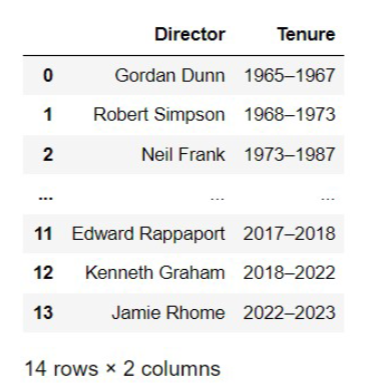We would like to merge storms with directors to produce a DataFrame with the same information as storms plus one additional column with the name of the director who was leading the NHC at the time of each storm. However, when we try to merge with the command shown below, Python fails to produce the desired DataFrame.

directors.merge(storms, left_on="Tenure", right_on="Year")

Which of the following is a problem with our attempted merge? Select all that apply.

• We cannot merge these two DataFrames because they have two completely different sets of column names.

• We want to add information about the directors to storms, so we need to use storms as our left DataFrame. The command should start with storms.merge(directors).

• The directors DataFrame does not contain enough information to determine who was the director of the NHC at the time of each storm.

• The "Tenure" column of directors contains a different data type than the "Year" column of storms.

Answer: Option 3 and Option 4

Recall that left_df.merge(right_df, left_on='column_a', right_on='column_b') merges left_df to the right_df and specifies which columns from the DataFrame to use as keys by using left_on= and right_on=. This means that column_a becomes the key for the left DataFrame and column_b becomes the key for the right DataFrame. That means the column names do not need to be the same. The important part of this is 'column_a' and 'column_b' should be the same data type and contain the same information for the merge to be successful.

Option 4 is correct because the years are formatted differenntly in storms and in directors. In storms the column "Year" contains an int, which is the year, whereas in "Tenure" the column contains a string to represent a span of years. When we try to merge there is no overlap between values in these columns. There will actually be an error because we are trying to merge two columns of different types.

Option 3 is correct because the merge will fail to happen due to the error we see caused by the columns containing values with no overlap.

Option 1: Is incorrect because you can merge DataFrames with different column names using left_on and right_on.

Option 2: Is incorrect because regardless of the left or right DataFrames if done correctly they will merge together. This means the order of the DataFrames does not make an impact.

##### Difficulty: ⭐️⭐️⭐️

The average score on this problem was 61%.

## Problem 10

### Problem 10.1

Recall that all the named storms in storms occurred between 1965 and 2015, a fifty-year time period.

Below is a histogram and the code that produced it. Use it to answer the questions that follow.

    (storms.groupby(["Name", "Year"]).count()
.reset_index()
.plot(kind="hist", y="Year",
density=True, ec="w",
bins=np.arange(1965, 2020, 5)));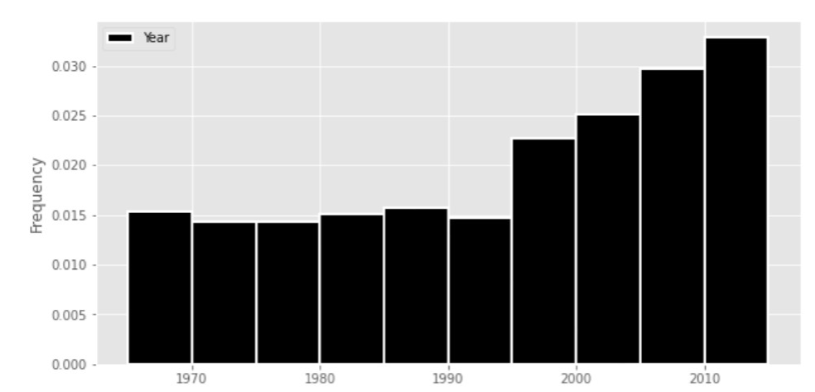Approximately (a) percent of named storms in this fifty-year time period occurred in 1995 or later. Give your answer to the nearest multiple of five.

We can find the percentage of named storms by using the fact: a histograms’ area is always normalized to 1. This means we can calculate the area of the rectangle, also known as width * height, to the left of 1995. The height, which is the frequency, is about 0.015 and the width is the difference between 1995 and 1965. This gives us: 1 - (1995 - 1965) * 0.015 = 0.55. Next to convert this to a percentage we multiply by 100, giving us: 0.55 * 100 = 55\%.

##### Difficulty: ⭐️⭐️⭐️

The average score on this problem was 52%.

### Problem 10.2

True or False? The line plot generated by the code below will have no downward-sloping segments after 1995.

    (storms.groupby(["Name", "Year"]).count()
.reset_index()
.groupby("Year").count()
.plot(kind="line", y="Name");
• True

• False

The previous histogram shows upward-sloping segments for each 5-year period after 1995; however, we are now ploting a line graph that shows a continuous timeline. Thus, there may be downward-sloping that happens within any 5-year periods.

##### Difficulty: ⭐️⭐️⭐️

The average score on this problem was 51%.

## Problem 11

### Problem 11.1

The code below defines a variable called month formed.

month_formed = (storms.groupby(["Name", "Year"]).min()
.reset_index()
.groupby("Month").count()
.get("Name"))

What is the data type of month formed?

• int

• str

• Series

• Dataframe

• None of these

It’s helpful to analyze the code piece by piece. The first part is doing .groupby(["Name", "Year"]).min(), which will index both "Name" and "Year" and find the minimum values in the DataFrame. We are still working with a DataFrame at this point. The next part .reset_index() makes "Name" and "Year" columns again. Again, this is a DataFrame. The next part .groupby("Month").count() makes "Month" the index and gets the count for each element in the DataFrame. Finally, .get("Name") isolates the "Name" column and returns to month_formed a series.

##### Difficulty: ⭐️⭐️

The average score on this problem was 81%.

### Problem 11.2

Which of the following expressions evaluates to the proportion of storms in our data set that were formed in August?

• month_formed.loc/month_formed.sum()

• month_formed.iloc/month_formed.sum()

• month_formed[month_formed.index == 8].shape/month_formed.sum()

• month_formed[month_formed.get("Month") == 8].shape/month_formed.sum()

Answer: month_formed.loc/month_formed.sum()

Option 1: Recall that August is the eigth month, so using .loc will find the label 8 in month_formed, which will be counts or the number of storms formed in August. Dividing the number of storms formed in August by the total number of storms formed will give us the proportion of storms that formed in August.

Option 2: It is important to realize that the months have become the index of month_formed, but that doesn’t necessarily mean that the index starts in January or that there have been storm during a month before August. For example if there were no storms in March then there would be no 3 in the index. Recall .iloc is indexing for whatever is in position 7, but because the index is not guaranteed we cannot be certain the .iloc will return August.

Option 3: The code: month_formed[month_formed.index == 8].shape will return 1. Finding the index at month 8 will give us August, but doing .shape gives us the number of rows in August, which should only be 1 because of groupby. This means that Option 3’s line of code will not give us the number of storms that formed in August, which makes it impossible to find the propotion.

Option 4: Remember that months_formed’s index is "Month". This means that there is no column "Month", so the code will error, meaning it cannot give us proportions of storms that formed in August.

##### Difficulty: ⭐️⭐️⭐️⭐️

The average score on this problem was 35%.

## Problem 12

### Problem 12.1

Hurricane forecasters use complex models to simulate hurricanes. Suppose a forecaster simulates 10,000 hurricanes and keeps track of the state where each hurricane made landfall in an array called landfalls. Each element of landfalls is a string, which is either the full name of a US state or the string "None" if the storm did not hit land in the simulation.

The forecaster wants to use the results of their simulation to estimate the probability that a given storm hits Georgia. Write one line of Python code that approximates this probability, using the data in landfalls.

Answer: np.count_nonzero(landfalls == "Georgia") / 10000

The probability would be the number of times the storm hit Georgia over the total number of simulated hurricanes, which is 10000. Since each element of landfills is a string, we can use np.count_nonzero() to give us all of the times the storm hits Georgia in the simulation. Recall np.count_nonzero() determines whether the elements are “truthy”, so if the String was equal to "Georgia" then it would be counted, and otherwise would be ignored. Then to calculate the probability we simply divide the number of times the storm hit Georgia by 10000.

##### Difficulty: ⭐️⭐️⭐️⭐️

The average score on this problem was 34%.

### Problem 12.2

Oh no, a hurricane is forming! Experts predict that the storm has a 45% chance of hitting Florida, a 25% chance of hitting Georgia, a 5% chance of hitting South Carolina, and a 25% chance of not making landfall at all.

Fast forward: the storm made landfall. Assuming the expert predictions were correct, what is the probability that the storm hit Georgia? Give your answer as a fully simplified fraction between 0 and 1.

Hint: The answer is not \frac{1}{4}, or 25%.